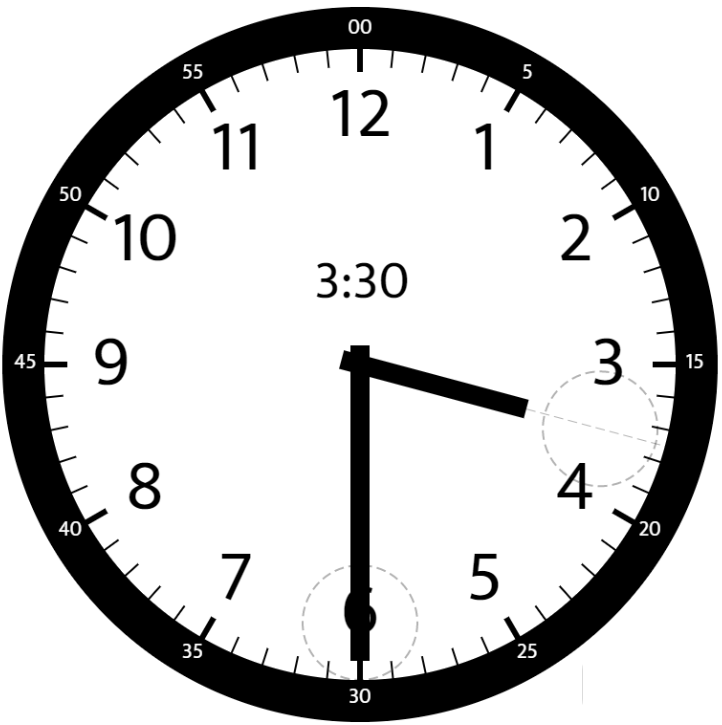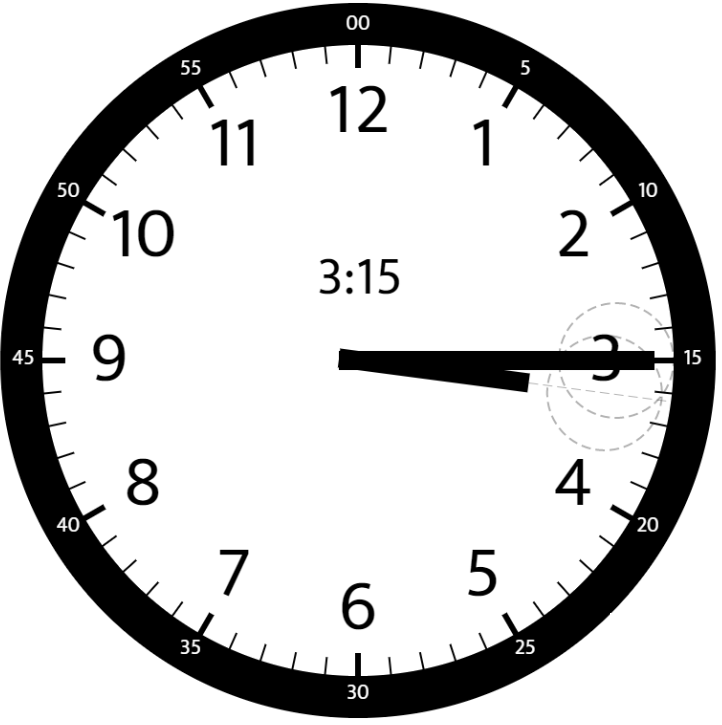## Saturday, July 25, 2020

### Problem Statement

Given two numbers, `hour` and `minutes`. Return the smaller angle (in degrees) formed between the `hour` and the `minute` hand.

Example 1:```Input: hour = 12, minutes = 30
Output: 165
```

Example 2:```Input: hour = 3, minutes = 30
Output: 75
```

Example 3:```Input: hour = 3, minutes = 15
Output: 7.5
```

Example 4:

```Input: hour = 4, minutes = 50
Output: 155
```

Example 5:

```Input: hour = 12, minutes = 0
Output: 0
```

Constraints:

• `1 <= hour <= 12`
• `0 <= minutes <= 59`
• Answers within `10^-5` of the actual value will be accepted as correct.

### Video Tutorial

You can find the detailed video tutorial here

### Thought Process

Purely a math problem. Calculate the clock's hour hand and minute hand separately.
• There are 60 minutes in 360 angle, so each minute is 6 degree in angle.
• Angle should be the absolute value of (minute angel - hour angle)
• Final angle should be min(angle, 360 - angle)

### Solutions

Time Complexity: O(1) since it's a math problem
Space Complexity: O(1) no extra space is used

## Friday, July 17, 2020

### Problem Statement

Given an array of integers `nums`.

A pair `(i,j)` is called good if `nums[i]` == `nums[j]` and `i` < `j`.

Return the number of good pairs.

Example 1:

```Input: nums = [1,2,3,1,1,3]
Output: 4
Explanation: There are 4 good pairs (0,3), (0,4), (3,4), (2,5) 0-indexed.
```

Example 2:

```Input: nums = [1,1,1,1]
Output: 6
Explanation: Each pair in the array are good.
```

Example 3:

```Input: nums = [1,2,3]
Output: 0
```

Constraints:

• `1 <= nums.length <= 100`
• `1 <= nums[i] <= 100`

### Video Tutorial

You can find the detailed video tutorial here

### Thought Process

Array is the most basic data structures and common solutions inclue
• Nested for loops O(N^2)
• Sort O(NlgN)
• Use extra space O(N)
This applies to this problem perfectly. The O(N^2) brute force solution is naive. We can also use a Map data structure (key is the number, value is the occurrence count) thus O(N). We can also sort the array and use this simple formula (also leetcode's hint) to calculate the good number pair.
Good pairs = N * (N-1) / 2 where N is how many duplicate numbers, this is from combination C(n^2), from n elements pick two

### Solutions

#### Use Map

Time Complexity: O(N), N is the array size
Space Complexity: O(N) since we use extra Map

#### Sort

Time Complexity: O(NlgN), N is the array size since we sort
Space Complexity: O(1) no extra space is used

• None

## Saturday, July 4, 2020

[Originally from the Post: System design interview: how to design a chat system (e.g., Facebook Messenger, WeChat or WhatsApp)]

Remind ourselves with the "READ MF!" methodology.

## Requirements

Simple text based search with "AND" or "OR" support. E.g., give me all the tweets that have words "Black" AND "Life" in it sorted by published time in descending order.

search(api_dev_key, search_terms, maximum_results_to_return, sort, page_token)

A follow up question would be sort those results in other orders, E.g., give me all the tweets that have words "Black" AND "Life" in it sorted by the most commented tweets in descending order.

## Estimation

• Storage: assuming twitter has 1B users, 50% of them post a tweet per day, each tweet is 140 char = 140 Byte. Each day, 1B * 0.5 * 280(roughly 300)Byte = 150GB data
• Write QPS = 1B user * 0.5 post a tweet per day / 86400 = 5787 QPS = 6000 QPS
• Query QPS = assuming 3x than write QPS = 18000 QPS
Write QPS in general we don't worry too much since it could be processed asynchronously. 18000 QPS for read is easy to handle as long as we have enough number of boxes and most of query would be returned via memory (not hitting disk).

## Key designs and terms

You can also divided the system as usual into three major layers, presentation, service and data layer.

A few key terminologies.

If the follow up question, we just need to build another index given the sort key or we could fetch the results on flight and sort (local optimal). Follow "Index -> Search -> Rank" steps.